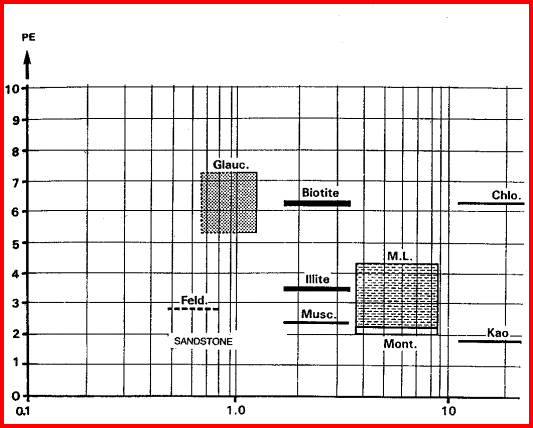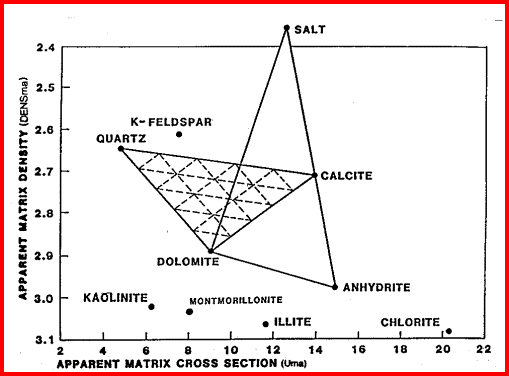SPECTRAL GAMMA RAY BASICS
There are two kinds of spectral gamma ray logs - the natural gamma ray spectral log and the induced gamma ray spectral log. The latter are sometimes called activation, geochemical (GST), or elemental capture spectroscopy (ECS) logs.

The induced gamma ray spectral log is an active device that emits gamma rays into the formation. Usually some form of pulsed neutron log is run in combination as well. The returning gamma rays and neutrons are filtered in a dozen or so energy windows, each corresponding to a particular element. The common elements identified are calcium (Ca), oxygen (O2), Chlorine (Cl), Iron (Fe), Carbon (C), Magnesium (Mg), Aluminum (Al) Silicon (Si), and a few trace elements that have large capture cross sections.

These are the elements found in most common minerals, such as quartz (SiO2), calcite (CaCO3), dolomite (Mg Ca CO3), shale (Si Al Fe O2). Along with thorium, potassium, and porosity derived from other logs, the elemental yields are transformed into mineral volumes by a least squares inversion, attempting to use up all the elements found with the least error. This process has an infinity of possible answers and sometimes fails to give rational results.

The natural gamma ray spectral log is a passive device that filters the natural gamma rays emitted by a formation into three components - potassium (K), uranium (U), and Thorium (Th). These logs are sometimes called KUT logs.

The thorium and potassium curves can be used together or in combination with PE, DENSma, or any other lithology indicator described earlier to determine two or three mineral models. Rather than repeat the equations here, the reader is encouraged to re-write the three mineral model given earlier for Uma-DENSma, substituting PE, K, or Th/K as desired. Crossplots shown below can be used to visualize many potential mineral combinations.

In most cases, shale intervals can be located from the total gamma ray, SP, or density neutron separation. In radioactive dolomites, this is not possible, since the GR responds to the uranium in the dolomite, the SP is flat due to the nature of the SP in carbonates, and the density neutron separation is due to the dolomite, not necessarily shale. The PE value of most shales is similar to dolomite, so PE is no help either. Radioactive dolomite looks exactly like shale on conventional logs.

In this situation, the natural gamma ray spectral log will provide information on the thorium, potassium, and uranium content of the rock. If thorium and/or potassium are low, and uranium is high, then the rock is not shale. Other data such as PE, density, and neutron are then used to define the porosity and  rock type. However, if thorium or potassium is high, with or without uranium, then some shale should be suspected, and accounted for.

Radioactive limestones also exist. These are less ambiguous as the density neutron separation is small and PE high. These characteristics are not shale indicators.

Sandstones also may be radioactive due to potassium in feldspar. Low uranium content and other log data should define this situation. The thorium curve is used as a shale indicator and the density neutron complex lithology crossplot model is used for porosity calculations.

Some reservoirs become radioactive at the well bore due to precipitation of uranium during oil production. This higher radioactivity compared to the original open hole logs can be confusing during workover or production logging operations. The uranium curve will help explain the problem.

The potassium curve is very useful in potash ore grade evaluation, and the uranium curve of course would be useful in the uranium mining and waste disposal businesses.

 Th/K Ratio in Clay High Kaolinite Chlorite Montemorillonite Illite Mica Glauconite Feldspar Low Potassium evaporateCROSSPLOTS FOR CLAY TYPING
Clay typing from well log data is usually accomplished by using crossplots of  potassium and thorium data from the gamma ray spectral log and the photo electric effect. If the shale is somewhat silty, the non-clay minerals will skew the results, requiring a detailed multi-mineral model calculation. Density, neutron, and PE data will also help sort out clay types.

The thorium/potassium ratio is another method of visually identifying some rocks. In decreasing order, the Th/K ratio list is as follows:

This ratio is illustrated graphically below.

A neat tool developed by Yalcin Pekiner for finding clay type from thorium potassium ratio can be found HERE.PE vs Th/K Ratio Crossplot for Clay Typing - note that most clay minerals do not represent data
"points" but are bands or blobs. Strict mathematical solutions are thus not likely to succeed,
but statistical approaches based on this crossplot are possible. X Axis is Th/K in ppm/%.PE vs Potassium Crossplot for  Clay Typing. If data points fall to the left of the clay points, the
data is from a silty shale or calcareous shale. Note the location of the potash minerals on the right
side of the plot. X Axis is K in %.Apparent Matrix DENSITY -  Photoelectric Cross Section of Shale
The matrix density (DFNSma) versus matrix cross section (Uma) crossplot is usually used to solve a 3-mineral model, but it can be used for clay typing by plotting data from shale intervals. If data is in porosity units, convert PHIDSH to density with equation 2. Calculate Ush from equation 3. Plot DENSSH on vertical axis and Ush on horizontal axis.

1: PHIt = (PHINSH + PHIDSH) / 2
2: DENSSH =  PHIDSH * KD1+ (1 - PHIDSH) * KD2
3: Ush = PESH * DENSSH / PHIt

Where:
KD1 = 1.00,  KD2 = 2.65 for Sandstone scale or 2.71 for Limestone scale
DENSSH = density log reading in shale (gm/cc)
PESH = effective photoelectric cross section in shale (barns/cm3)
PHINSH = neutron porosity in shale (fractional)
PHIDSH = density porosity in shale (fractional)
PHINSH = neutron porosity in shale (fractional)
USH = photoelectric absorption of shale (barns/cm3)Matrix Density vs Matrix Cross Section Crossplot for Lithology. If plotted data points fall
above the clay points, the data is from a silty shale or a calcareous shale.

Page Views ---- Since 01 Jan 2015
Copyright 2023 by Accessible Petrophysics Ltd.
CPH Logo, "CPH", "CPH Gold Member", "CPH Platinum Member", "Crain's Rules", "Meta/Log", "Computer-Ready-Math", "Petro/Fusion Scripts" are Trademarks of the Author